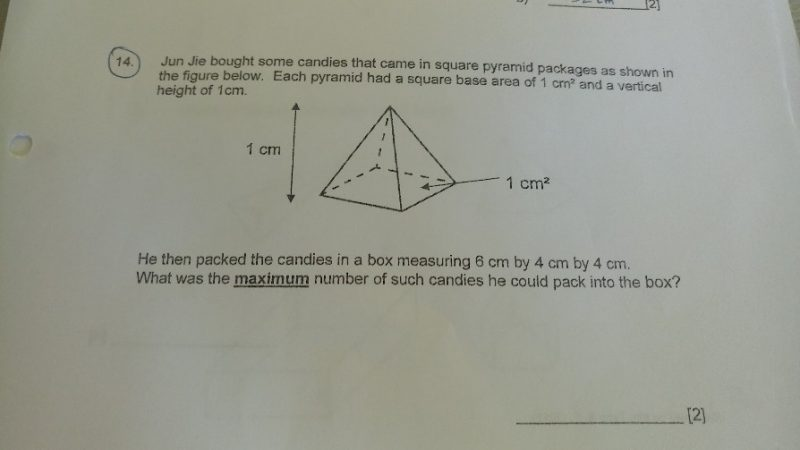# QuestionPls help. Its a nets question

This is a Volume question.

1.  Box. Length = 6, breadth = 4

First layer can put 6 × 4 = 24

Inverted first layer 6 × 4 = 24.

Total = 24 + 24  =48

2. Total 4 layers.

48 × 4 = 192 candles.

😊

0 Replies 0 Likes